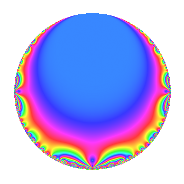# Properties

 Label 1155.2.a.gLevel 1155 Weight 2 Character orbit 1155.a Self dual Yes Analytic conductor 9.223 Analytic rank 1 Dimension 1 CM No Inner twists 1

# Learn more about

## Newspace parameters

 Level: $$N$$ = $$1155 = 3 \cdot 5 \cdot 7 \cdot 11$$ Weight: $$k$$ = $$2$$ Character orbit: $$[\chi]$$ = 1155.a (trivial)

## Newform invariants

 Self dual: Yes Analytic conductor: $$9.22272143346$$ Analytic rank: $$1$$ Dimension: $$1$$ Coefficient field: $$\mathbb{Q}$$ Coefficient ring: $$\mathbb{Z}$$ Coefficient ring index: $$1$$ Fricke sign: $$1$$ Sato-Tate group: $\mathrm{SU}(2)$

## $q$-expansion

 $$f(q)$$ $$=$$ $$q$$ $$\mathstrut -\mathstrut q^{3}$$ $$\mathstrut -\mathstrut 2q^{4}$$ $$\mathstrut -\mathstrut q^{5}$$ $$\mathstrut +\mathstrut q^{7}$$ $$\mathstrut +\mathstrut q^{9}$$ $$\mathstrut +\mathstrut O(q^{10})$$ $$q$$ $$\mathstrut -\mathstrut q^{3}$$ $$\mathstrut -\mathstrut 2q^{4}$$ $$\mathstrut -\mathstrut q^{5}$$ $$\mathstrut +\mathstrut q^{7}$$ $$\mathstrut +\mathstrut q^{9}$$ $$\mathstrut +\mathstrut q^{11}$$ $$\mathstrut +\mathstrut 2q^{12}$$ $$\mathstrut +\mathstrut q^{15}$$ $$\mathstrut +\mathstrut 4q^{16}$$ $$\mathstrut +\mathstrut 3q^{17}$$ $$\mathstrut -\mathstrut 3q^{19}$$ $$\mathstrut +\mathstrut 2q^{20}$$ $$\mathstrut -\mathstrut q^{21}$$ $$\mathstrut -\mathstrut q^{23}$$ $$\mathstrut +\mathstrut q^{25}$$ $$\mathstrut -\mathstrut q^{27}$$ $$\mathstrut -\mathstrut 2q^{28}$$ $$\mathstrut -\mathstrut 7q^{29}$$ $$\mathstrut +\mathstrut 6q^{31}$$ $$\mathstrut -\mathstrut q^{33}$$ $$\mathstrut -\mathstrut q^{35}$$ $$\mathstrut -\mathstrut 2q^{36}$$ $$\mathstrut -\mathstrut 8q^{37}$$ $$\mathstrut -\mathstrut 2q^{41}$$ $$\mathstrut -\mathstrut 5q^{43}$$ $$\mathstrut -\mathstrut 2q^{44}$$ $$\mathstrut -\mathstrut q^{45}$$ $$\mathstrut -\mathstrut 6q^{47}$$ $$\mathstrut -\mathstrut 4q^{48}$$ $$\mathstrut +\mathstrut q^{49}$$ $$\mathstrut -\mathstrut 3q^{51}$$ $$\mathstrut -\mathstrut 3q^{53}$$ $$\mathstrut -\mathstrut q^{55}$$ $$\mathstrut +\mathstrut 3q^{57}$$ $$\mathstrut -\mathstrut 5q^{59}$$ $$\mathstrut -\mathstrut 2q^{60}$$ $$\mathstrut -\mathstrut 7q^{61}$$ $$\mathstrut +\mathstrut q^{63}$$ $$\mathstrut -\mathstrut 8q^{64}$$ $$\mathstrut +\mathstrut 6q^{67}$$ $$\mathstrut -\mathstrut 6q^{68}$$ $$\mathstrut +\mathstrut q^{69}$$ $$\mathstrut -\mathstrut 10q^{71}$$ $$\mathstrut +\mathstrut 12q^{73}$$ $$\mathstrut -\mathstrut q^{75}$$ $$\mathstrut +\mathstrut 6q^{76}$$ $$\mathstrut +\mathstrut q^{77}$$ $$\mathstrut -\mathstrut 16q^{79}$$ $$\mathstrut -\mathstrut 4q^{80}$$ $$\mathstrut +\mathstrut q^{81}$$ $$\mathstrut -\mathstrut 3q^{83}$$ $$\mathstrut +\mathstrut 2q^{84}$$ $$\mathstrut -\mathstrut 3q^{85}$$ $$\mathstrut +\mathstrut 7q^{87}$$ $$\mathstrut +\mathstrut 3q^{89}$$ $$\mathstrut +\mathstrut 2q^{92}$$ $$\mathstrut -\mathstrut 6q^{93}$$ $$\mathstrut +\mathstrut 3q^{95}$$ $$\mathstrut -\mathstrut q^{97}$$ $$\mathstrut +\mathstrut q^{99}$$ $$\mathstrut +\mathstrut O(q^{100})$$

## Embeddings

For each embedding $$\iota_m$$ of the coefficient field, the values $$\iota_m(a_n)$$ are shown below.

For more information on an embedded modular form you can click on its label.

Label $$\iota_m(\nu)$$ $$a_{2}$$ $$a_{3}$$ $$a_{4}$$ $$a_{5}$$ $$a_{6}$$ $$a_{7}$$ $$a_{8}$$ $$a_{9}$$ $$a_{10}$$
1.1
 0
0 −1.00000 −2.00000 −1.00000 0 1.00000 0 1.00000 0
 $$n$$: e.g. 2-40 or 990-1000 Significant digits: Format: Complex embeddings Normalized embeddings Satake parameters Satake angles

## Inner twists

This newform does not admit any (nontrivial) inner twists.

## Atkin-Lehner signs

$$p$$ Sign
$$3$$ $$1$$
$$5$$ $$1$$
$$7$$ $$-1$$
$$11$$ $$-1$$

## Hecke kernels

This newform can be constructed as the intersection of the kernels of the following linear operators acting on $$S_{2}^{\mathrm{new}}(\Gamma_0(1155))$$:

 $$T_{2}$$ $$T_{13}$$ $$T_{17}$$ $$\mathstrut -\mathstrut 3$$